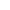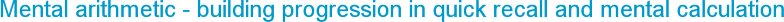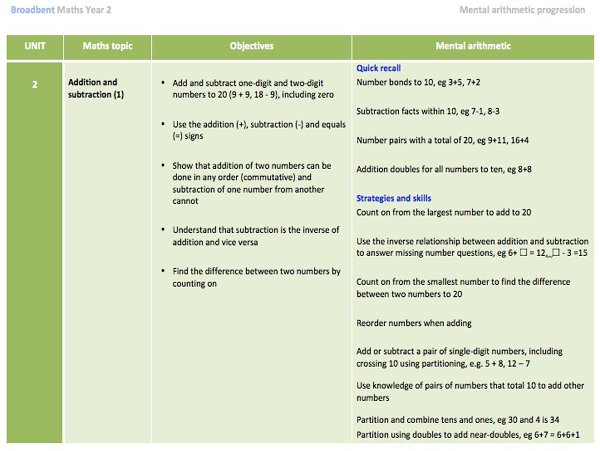###Saturday, 13 June 2015
Mental arithmetic has two distinct elements to consider when planning and teaching it as part of the maths curriculum. Firstly it involves the quick recall of number facts and secondly the use of mental methods or strategies to carry out mental calculations. Carefully thought through lines of progression are important for both these elements.

Quick recall

This is possibly the traditional view of mental arithmetic skills – the ability to quickly answer multiplication tables or know by heart the number bonds to 20. It is obviously important and without committing these number facts to memory it becomes increasingly difficult and inhibiting when carrying out calculations or problems.

The main reason for learning these number facts is as a springboard for answering questions that involve using those facts. Knowing that 6+7=13 makes calculating the answer to 60+70 or 56+7 or 0.6+0.7 just so much easier. Memorising and knowing the fact that 8x7=56 extinguishes the initial angst that children (and adults) feel when faced with calculating the cost of, for example, 8 yoghurts costing 27p each. Confidence plays a huge part in being able to calculate mentally, and not knowing number facts is a first and often pivotal knock of that confidence.

Once armed with this quick recall (and this takes practice and then more practice), it then frees you up to look at what the problem is asking, play with the number facts, use them to help answer the problem and use the relationships between the four operations confidently. It gives you that ‘comfort’ with number, which is so important when developing the ability to think mathematically.

Strategies and skills

As soon as key facts are learnt children are then able to use them to help calculate mentally with appropriate strategies and skills. This is where careful progression and questioning is important. Once a child knows, for example, that double 4 is 8 they need to be shown how useful this is for answering other questions. Through the use of models such as multilink, 10-frames or numicon they can be shown that 4+5 is just 1 more than double 4, and so they can begin to learn another fact, 4+5, to use for other calculations.

It is the ability to partition numbers confidently that seems key to the development of mental arithmetic skills. From an early age children need to be encouraged to play with numbers, breaking them apart and putting them together in different ways. Equivalence and transformation (what’s the same and what is different…) are the processes that are central to this and contribute to children learning with understanding. Through this they can spot connections between the different strands of mathematics.

Exploring the ‘Story of 6’, for example, allows young children to use models to find ways of partitioning 6 into 5+1, 4+2, 3+3, 2+4 and 1+5. This can be related to 6-1=5, 6-2=4, 6-3=3, 6-4=2 and 6-5=1. That is ten facts, all connected to and derived from the story of 6. Those ten facts can go on to help children use strategies that involve partitioning in different ways. For example, 60-22 could be answered by subtracting 20 from 60 and then subtracting another 2. This is relatively easy if you know that 6-2=4 and understand place value and equivalent values when making numbers 10 times bigger.

This ability to confidently calculate mentally is a feature of a mastery approach to teaching - without this ability it makes the next steps on the mathematical journey rather precarious.

It means that careful thought needs to go into the lines of progression for each strand, making sure that each step builds on the previous and is connected to others where possible.

The current Programmes of Study have also raised expectation, so, for example, the quick recall of all multiplication facts to 12x12 is now a target for the end of Y4.

This is a sample unit from Year 2 to show the type of content.Progression of mental arithmetic skills

Broadbent Maths Menu user schools can download the mental arithmetic progression documents, with ‘Quick Recall’ and ‘Strategies and skills’ objectives to focus on, related to each unit for Y1-Y6.

You can use this to help plan a programme of specific mental arithmetic activities and practice tasks so that children develop a comfort and confidence with number.

Related articles:

Making the most of the whole class maths starter
Planning mental starters

Make your own interactive maths kit
A bag of maths resources for each child.